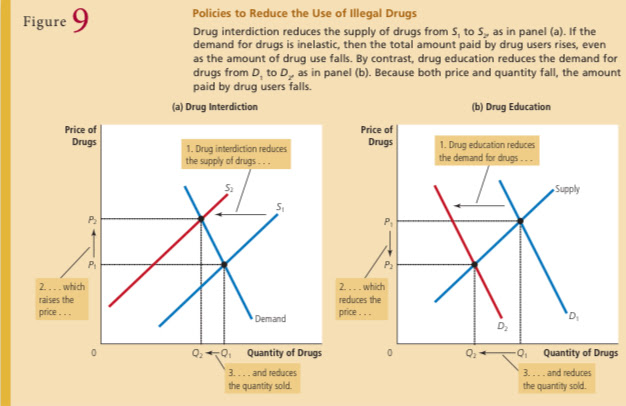# 5.1 The Elasticity of Demand

• Elasticity- a measure of the responsiveness of quantity demanded or quantity supplied to a change in one of its determinants

• Price elasticity of demand- a measure of how much the quantity demanded of a good responds to a change in the price of that good, computed as the percentage change in quantity demanded divided by the percentage change in price

• Inelastic demand- when the quantity demanded responds only slightly to changes in the price.

• Factors that influence the price elasticity of demand

• Availability of close substitutes

• Necessities versus Luxuries

• Definition of the Market

• Time Horizon

Computing the Price Elasticity of Demand:

• Price elasticity of demand= Percentage change in quantity demanded/

Percentage change in price (FORMULA)

The Midpoint Method: A Better Way to Calculate Percentage Changes and Elasticities:

• Price elasticity of demand= (Q2 Q1 ) / [(Q2 Q1 ) / 2] /

(P2 P1 ) / [(P2  P1 ) / 2] (FORMULA)

The Variety of Demand Curves:

• Demand is considered elastic when the elasticity is greater than 1

• Which means the quantity moves proportionately more than the price.

• If the elasticity is exactly 1, the quantity moves the same amount proportionately as the price

• Demand is said to have unit elasticity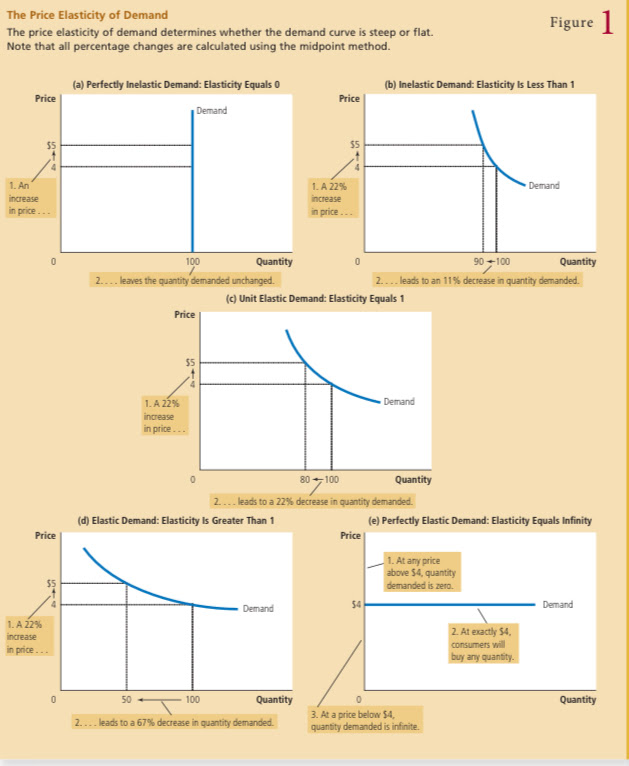Total Revenue and the Price Elasticity of Demand:

• Total revenue- the amount paid by buyers and received by sellers of a good, computed as the price elasticity of demand rise of the good times the quantity soldElasticity and Total Revenue along a Linear Demand Curve:

• (In a demanding schedule) The slope of a linear demand curve is constant, but its elasticity is not.

• At points with a low price and high quantity, the demand curve is inelastic. At points with a high price and low quantity, the demand curve is elastic.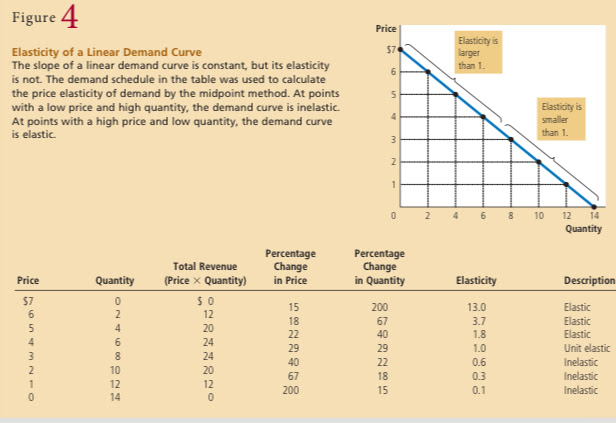Other Demand Elasticities:

• Other elasticities are used to describe the behavior of buyers in a market.

• Income elasticity of demand- the measure of how much the quantity demanded changes as consumer income changes.

• The cross-price elasticity of demand- the measure of how much the quantity demanded of one good responds to a change in the price of another good.

# 5.2 The Elasticity of Supply

The Price Elasticity of Supply and Its Determinants:

• Price elasticity of supply- the measure of how much the quantity supplied responds to changes in the price

• The supply of a good is said to be elastic if the quantity supplied response substantially to changes in the price.

• Supply is said to be inelastic if the quantity supplied responds only slightly to changes in the price

Computing the Price Elasticity of Supply:

• Price elasticity of supply = Percentage change in quantity supplied/

Percentage change in price

The Variety of Supply Curves:

• In some markets, the elasticity of supply is not constant

• Can vary over the supply curve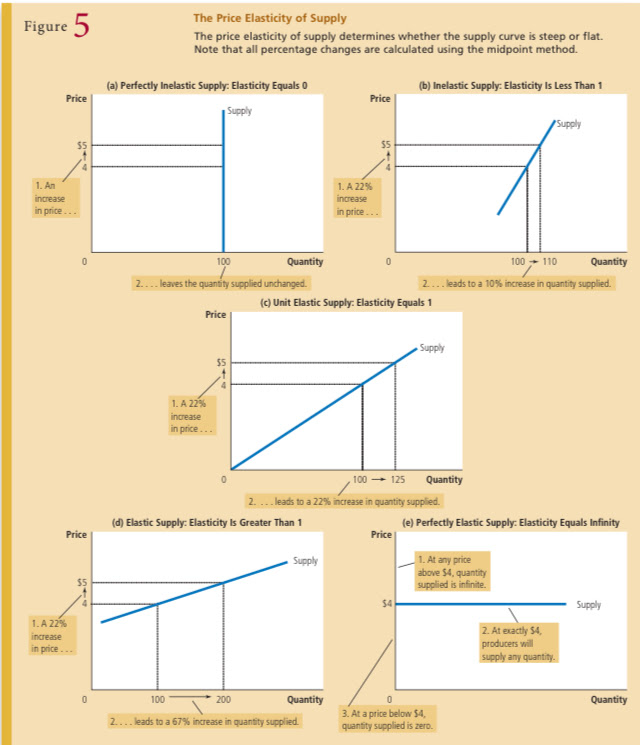# 5.3: Three Applications of Supply, Demand, and Elasticity

Can Good News for Farming Be Bad News for Farmers?:

• In one scenario, you are a farmer who recently heard about a new discovery that raises the amount farmers can produce from each acre of land by 20 percent.

• In order to see if this discovery makes you better off or worse off than you were before, we can use three steps to answer such a question mentioned in Chapter 4.

• First, we examine whether the supply or demand curve shifts.

• Second, we consider in which direction the curve shifts.

• Third, we use the supply-and-demand diagram to see how the market equilibrium changes.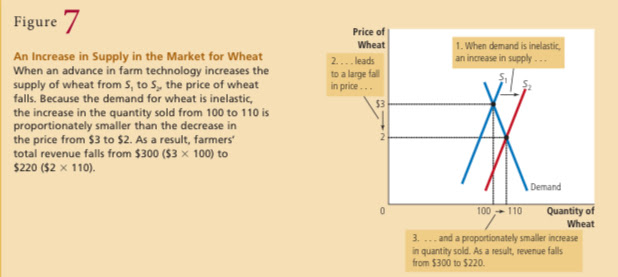Why Did OPEC Fail to Keep the Price of Oil High?:

• Oil was the cause of the most disruptive events in the world’s economy

• The Organization of Petroleum Exporting Countries (OPEC) decided to raise the world price of oil to increase their incomes.

• These countries accomplished this goal by jointly reducing the amount of oil they supplied.

• It was difficult to maintain the high price

• When the supply of oil falls, the response depends on the time horizon. In the short run, supply and demand are relatively inelastic

• In the long run, supply and demand are relatively elastic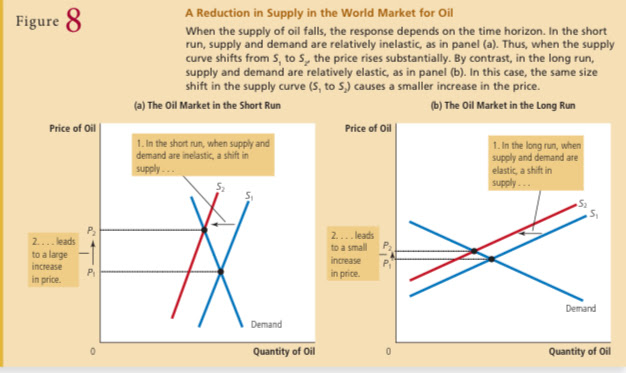• They’ve come to the conclusion that OPEC’s coordinated reduction in supply

proved less profitable in the long run. The cartel learned that raising prices is easier in the short run than in the long run.

Does Drug Interdiction Increase or Decrease Drug-Related Crime?:

• Illegal drugs such as heroin, cocaine, ecstasy, and crack are followed by consequences

• Drug dependence can ruin the lives of drug users and their families

• Drug addicts often turn to robbery and other violent crimes to obtain the money needed to support their habit

• The U.S. government has spent billions of dollars each year to discourage the use of illegal drugs

• Though the purpose of drug interdiction is meant to reduce the use of drugs, it mainly impacts the buyers rather than the sellers

• The demand for drugs—the amount buyers want at any given price—is not changed.

• Drug interdiction could increase drug-related crime.